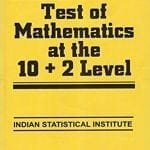How Cheenta works to ensure student success?
Explore the Back-Story

# Test of Mathematics Solution Subjective 38 - When 30 divides a primeTest of Mathematics Solution Subjective 38 (from ISI Entrance). The book, Test of Mathematics at 10+2 Level is Published by East West Press. This problem book is indispensable for the preparation of I.S.I. B.Stat and B.Math Entrance.

## Problem

Show that if a prime number p is divided by 30, the remainder is either prime or 1.

## Discussion

Suppose p = 30Q + R

(here p is the prime, Q and R are quotient and remainders respectively when p is divided by 30).

For all primes less than 30, Q = 0 and R=p. So that satisfies the claim of this problem.

If p > 30, suppose the remainder R is composite (not a prime). Since R 6 and N > 6 then MN (=R) > 36 > 30. But R < 30. So both M and N cannot exceed 6. Suppose M < 6. Then M must be divisible by 2, 3, or 5. We consider the case when M is divisible by 2 (other cases are analogous).

Suppose M = 2M'

R = MN = 2M'N

p = 30Q + 2M'N

But then the right hand side is divisible by 2. Hence the left hand side is also divisible by 2. But that is not possible as p is a prime larger than 30.

Hence R cannot be composite. This implies R is either a prime or 1.Test of Mathematics Solution Subjective 38 (from ISI Entrance). The book, Test of Mathematics at 10+2 Level is Published by East West Press. This problem book is indispensable for the preparation of I.S.I. B.Stat and B.Math Entrance.

## Problem

Show that if a prime number p is divided by 30, the remainder is either prime or 1.

## Discussion

Suppose p = 30Q + R

(here p is the prime, Q and R are quotient and remainders respectively when p is divided by 30).

For all primes less than 30, Q = 0 and R=p. So that satisfies the claim of this problem.

If p > 30, suppose the remainder R is composite (not a prime). Since R 6 and N > 6 then MN (=R) > 36 > 30. But R < 30. So both M and N cannot exceed 6. Suppose M < 6. Then M must be divisible by 2, 3, or 5. We consider the case when M is divisible by 2 (other cases are analogous).

Suppose M = 2M'

R = MN = 2M'N

p = 30Q + 2M'N

But then the right hand side is divisible by 2. Hence the left hand side is also divisible by 2. But that is not possible as p is a prime larger than 30.

Hence R cannot be composite. This implies R is either a prime or 1.

### Knowledge Partner